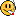## Arbitrary (and unjustified) cut-off of r² [RSABE / ABEL]

Dear Detlew & AB!

❝ Not to calculate the AUC(0-inf) values if the fit of the terminal part of the concentration-time curves had an r2 value less than 80% is at least statistically not very sound, not to say nonsense IMHO.

Yes! Explained variance (sloppy: information) in regression (strongly!) depends on the sample size. See here and a rather lengthy thread of 2002 at David Bourne’s PKPD-list.
Critical values of $$\small{r}$$ according to Odeh (1982)1 (and modified for $$\small{r^2}$$); one sided, 5%:$$\small{\begin{array}{rcc} n & r & r^2\\\hline 3 & 0.9877 & 0.9755\\ 4 & 0.9000 & 0.8100\\ 5 & 0.805\phantom{0} & 0.6486\\ 6 & 0.729\phantom{0} & 0.5319\\ 7 & 0.669\phantom{0} & 0.4481\\ 8 & 0.621\phantom{0} & 0.3863\\ 9 & 0.582\phantom{0} & 0.3390\\ 10 & 0.549\phantom{0} & 0.3018\\ 11 & 0.521\phantom{0} & 0.2719\\ 12 & 0.497\phantom{0} & 0.2473\\ 13 & 0.476\phantom{0} & 0.2267\\ 14 & 0.457\phantom{0} & 0.2093\\ 15 & 0.441\phantom{0} & 0.1944\\\hline \end{array}}$$ In other words, an $$\small{r^2}$$ of 0.6486 from five data points denotes the same ‘quality of fit’ than an $$\small{r^2}$$ of 0.9755 from three. Searching the forum I get the impression that you (AB) are not alone with a cut-off of 0.80. Justification: nil. Maybe there is some copypasting going on? If you really want to use a cut-off (which I don’t recommend and is not required in any GL) take the number of data points into account. I strongly suggest to revise your SOP.
BTW, visual inspection of fits is mandatory (see there with references). Don’t trust in numbers alone. A classical example is Anscombe’s quartet.2All data sets: $$\small{\bar{x}=9.0,\,s_x^2=11,\,\bar{y}=7.5,\,s_x^2=4.1\rightarrow\widehat{y}=3+0.5\cdot x,\,R_{yx}^2=0.82\ldots}$$

1. Odeh RE. Critical values of the sample product-moment correlation coefficient in the bivariate distribution. Commun Statist–Simula Computa. 1982; 11(1): 1–26. doi:10.1080/03610918208812243.
2. Anscombe FJ. Graphs in statistical analysis. Am Stat. 1973; 27: 17–21. doi:10.2307/2682899.

Dif-tor heh smusma 🖖🏼 Довге життя Україна!Helmut SchützThe quality of responses received is directly proportional to the quality of the question asked. 🚮
Science QuotesIng. Helmut Schütz